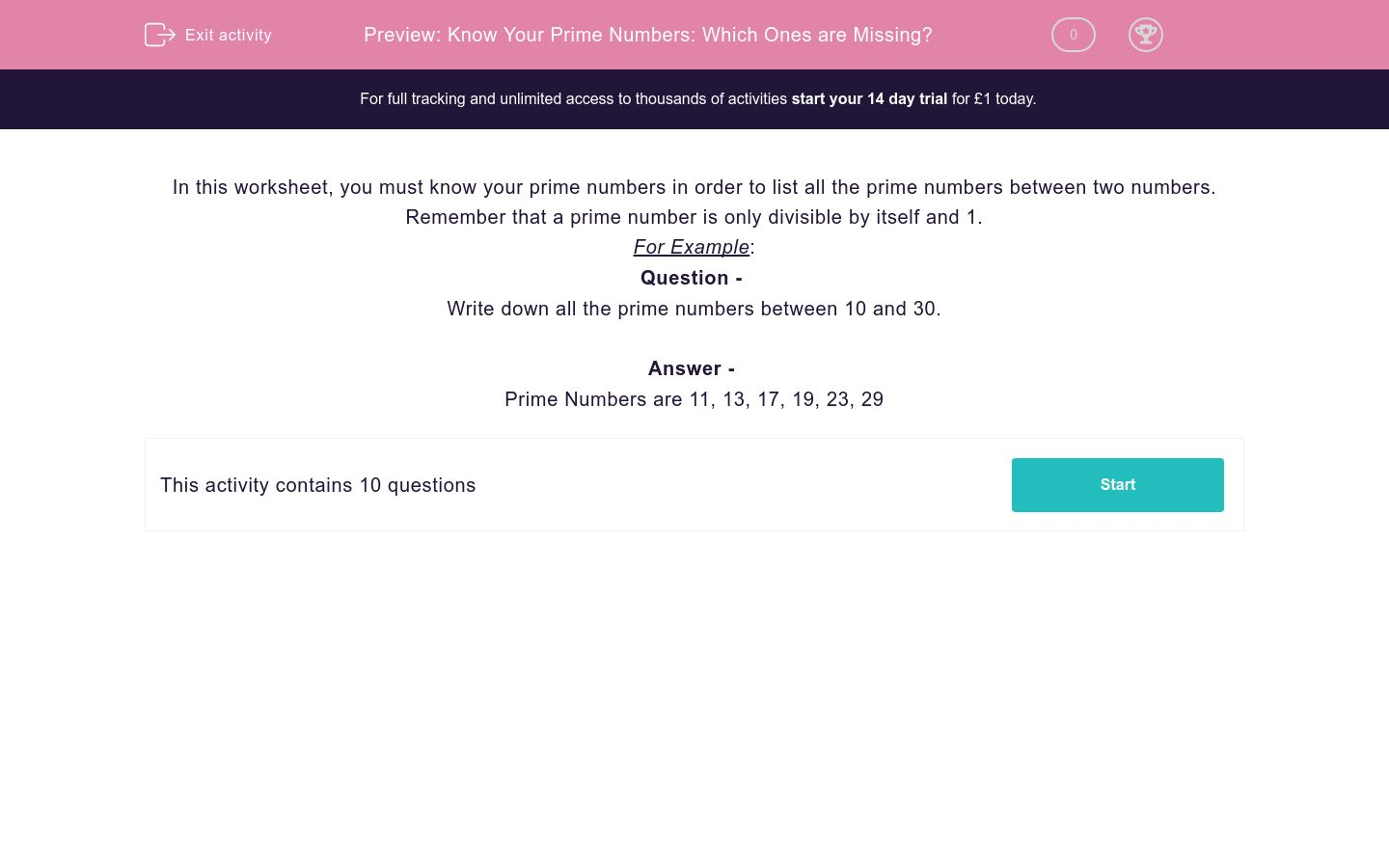# Know Your Prime Numbers: Which Ones are Missing?

In this worksheet, students identify all prime numbers between two given numbers.Key stage:  KS 2

Curriculum topic:   Maths and Numerical Reasoning

Curriculum subtopic:   Special Numbers

Difficulty level:### QUESTION 1 of 10

In this worksheet, you must know your prime numbers in order to list all the prime numbers between two numbers.

Remember that a prime number is only divisible by itself and 1.

For Example:

Question -
Write down all the prime numbers between 10 and 30.

Prime Numbers are 11, 13, 17, 19, 23, 29

Select all the prime numbers between 30 and 50.

30

31

33

37

41

43

45

47

Select all the prime numbers between 10 and 30.

11

13

16

17

19

23

27

29

Write down all the prime numbers between 70 and 90 (in ascending order) in the boxes below.

 Prime Numbers 1st 2nd 3rd 4th 5th

Write down all the prime numbers between 60 and 80 (in ascending order) in the boxes below.

 Prime Numbers 1st 2nd 3rd 4th 5th

Write down all the prime numbers between 40 and 60 (in ascending order) in the boxes below.

 Prime Numbers 1st 2nd 3rd 4th 5th

Write down all the prime numbers between 50 and 70 (in ascending order) in the boxes below.

 Prime Numbers 1st 2nd 3rd 4th

Write down all the prime numbers between 80 and 100 (in ascending order) in the boxes below.

 Prime Numbers 1st 2nd 3rd

Write down all the prime numbers between 50 and 70 (in ascending order) in the boxes below.

 Prime Numbers 1st 2nd 3rd 4th

Write down all the prime numbers between 20 and 40 (in ascending order) in the boxes below.

 Prime Numbers 1st 2nd 3rd 4th

Write down all the prime numbers between 20 and 45 (in ascending order) in the boxes below.

 Prime Numbers 1st 2nd 3rd 4th 5th 6th
• Question 1

Select all the prime numbers between 30 and 50.

31
37
41
43
47
• Question 2

Select all the prime numbers between 10 and 30.

11
13
17
19
23
29
• Question 3

Write down all the prime numbers between 70 and 90 (in ascending order) in the boxes below.

 Prime Numbers 1st 2nd 3rd 4th 5th
• Question 4

Write down all the prime numbers between 60 and 80 (in ascending order) in the boxes below.

 Prime Numbers 1st 2nd 3rd 4th 5th
• Question 5

Write down all the prime numbers between 40 and 60 (in ascending order) in the boxes below.

 Prime Numbers 1st 2nd 3rd 4th 5th
• Question 6

Write down all the prime numbers between 50 and 70 (in ascending order) in the boxes below.

 Prime Numbers 1st 2nd 3rd 4th
• Question 7

Write down all the prime numbers between 80 and 100 (in ascending order) in the boxes below.

 Prime Numbers 1st 2nd 3rd
• Question 8

Write down all the prime numbers between 50 and 70 (in ascending order) in the boxes below.

 Prime Numbers 1st 2nd 3rd 4th
• Question 9

Write down all the prime numbers between 20 and 40 (in ascending order) in the boxes below.

 Prime Numbers 1st 2nd 3rd 4th
• Question 10

Write down all the prime numbers between 20 and 45 (in ascending order) in the boxes below.

 Prime Numbers 1st 2nd 3rd 4th 5th 6th
EDDIE SAYS
These are the numbers with exactly two factors.
---- OR ----

Sign up for a £1 trial so you can track and measure your child's progress on this activity.

### What is EdPlace?

We're your National Curriculum aligned online education content provider helping each child succeed in English, maths and science from year 1 to GCSE. With an EdPlace account you’ll be able to track and measure progress, helping each child achieve their best. We build confidence and attainment by personalising each child’s learning at a level that suits them.

Get started